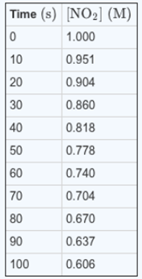# Problem: Consider the following reaction: NO2(g) → NO(g) + 1/2O2(g) The following data were collected for the concentration of NO2 as a function of time:A. What is the average rate of the reaction between 10 and 20 s?B. What is the average rate of the reaction between 50 and 60 s?C. What is the rate of formation of O2 between 50 and 60 s?

###### FREE Expert Solution

We are being asked to determine the average of the reaction for the reaction:

NO2(g) → NO(g) + 1/2O2(g)

Recall that for a reaction aA  bB, the rate of a reaction is given by:

$\overline{){\mathbf{Rate}}{\mathbf{=}}{\mathbf{-}}\frac{\mathbf{1}}{\mathbf{a}}\frac{\mathbf{\Delta }\mathbf{\left[}\mathbf{A}\mathbf{\right]}}{\mathbf{\Delta t}}{\mathbf{=}}\frac{\mathbf{1}}{\mathbf{b}}\frac{\mathbf{\Delta }\mathbf{\left[}\mathbf{B}\mathbf{\right]}}{\mathbf{\Delta t}}}$

where:

Δ[A] = change in concentration of reactants or products (in mol/L or M), [A]final – [A]initial

Δt = change in time, tfinal – tinitial

Since NO2 is a reactant, the rate with respect to NO2 is negative (–) since we’re losing reactants.

100% (424 ratings)###### Problem Details

Consider the following reaction: NO2(g) → NO(g) + 1/2O2(g) The following data were collected for the concentration of NO2 as a function of time:

A. What is the average rate of the reaction between 10 and 20 s?

B. What is the average rate of the reaction between 50 and 60 s?

C. What is the rate of formation of O2 between 50 and 60 s?Frequently Asked Questions

What scientific concept do you need to know in order to solve this problem?

Our tutors have indicated that to solve this problem you will need to apply the Average Rate of Reaction concept. You can view video lessons to learn Average Rate of Reaction. Or if you need more Average Rate of Reaction practice, you can also practice Average Rate of Reaction practice problems.